# Private Ads prediction with DP-SGD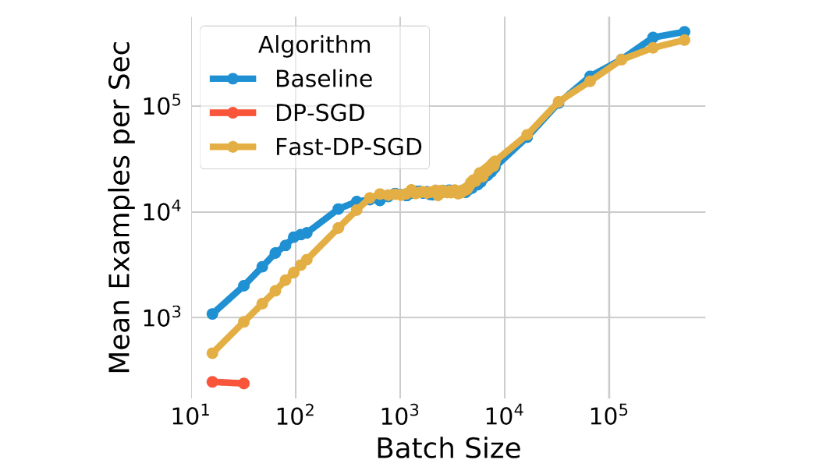Ad technology providers widely use machine learning (ML) models to predict and present users with the most relevant ads, and to measure the effectiveness of those ads. With increasing focus on online privacy, there’s an opportunity to identify ML algorithms that have better privacy-utility trade-offs. Differential privacy (DP) has emerged as a popular framework for developing ML algorithms responsibly with provable privacy guarantees. It has been extensively studied in the privacy literature, deployed in industrial applications and employed by the U.S. Census. Intuitively, the DP framework enables ML models to learn population-wide properties, while protecting user-level information.

When training ML models, algorithms take a dataset as their input and produce a trained model as their output. Stochastic gradient descent (SGD) is a commonly used non-private training algorithm that computes the average gradient from a random subset of examples (called a mini-batch), and uses it to indicate the direction towards which the model should move to fit that mini-batch. The most widely used DP training algorithm in deep learning is an extension of SGD called DP stochastic gradient descent (DP-SGD).

DP-SGD includes two additional steps: 1) before averaging, the gradient of each example is norm-clipped if the L2 norm of the gradient exceeds a predefined threshold; and 2) Gaussian noise is added to the average gradient before updating the model. DP-SGD can be adapted to any existing deep learning pipeline with minimal changes by replacing the optimizer, such as SGD or Adam, with their DP variants. However, applying DP-SGD in practice could lead to a significant loss of model utility (i.e., accuracy) with large computational overheads. As a result, various research attempts to apply DP-SGD training on more practical, large-scale deep learning problems. Recent studies have also shown promising DP training results on computer vision and natural language processing problems.

In “Private Ad Modeling with DP-SGD”, we present a systematic study of DP-SGD training on ads modeling problems, which pose unique challenges compared to vision and language tasks. Ads datasets often have a high imbalance between data classes, and consist of categorical features with large numbers of unique values, leading to models that have large embedding layers and highly sparse gradient updates. With this study, we demonstrate that DP-SGD allows ad prediction models to be trained privately with a much smaller utility gap than previously expected, even in the high privacy regime. Moreover, we demonstrate that with proper implementation, the computation and memory overhead of DP-SGD training can be significantly reduced.

## Evaluation

We evaluate private training using three ads prediction tasks: (1) predicting the click-through rate (pCTR) for an ad, (2) predicting the conversion rate (pCVR) for an ad after a click, and 3) predicting the expected number of conversions (pConvs) after an ad click. For pCTR, we use the Criteo dataset, which is a widely used public benchmark for pCTR models. We evaluate pCVR and pConvs using internal Google datasets. pCTR and pCVR are binary classification problems trained with the binary cross entropy loss and we report the test AUC loss (i.e., 1 - AUC). pConvs is a regression problem trained with Poisson log loss (PLL) and we report the test PLL.

For each task, we evaluate the privacy-utility trade-off of DP-SGD by the relative increase in the loss of privately trained models under various privacy budgets (i.e., privacy loss). The privacy budget is characterized by a scalar ε, where a lower ε indicates higher privacy. To measure the utility gap between private and non-private training, we compute the relative increase in loss compared to the non-private model (equivalent to ε = ∞). Our main observation is that on all three common ad prediction tasks, the relative loss increase could be made much smaller than previously expected, even for very high privacy (e.g., ε <= 1) regimes.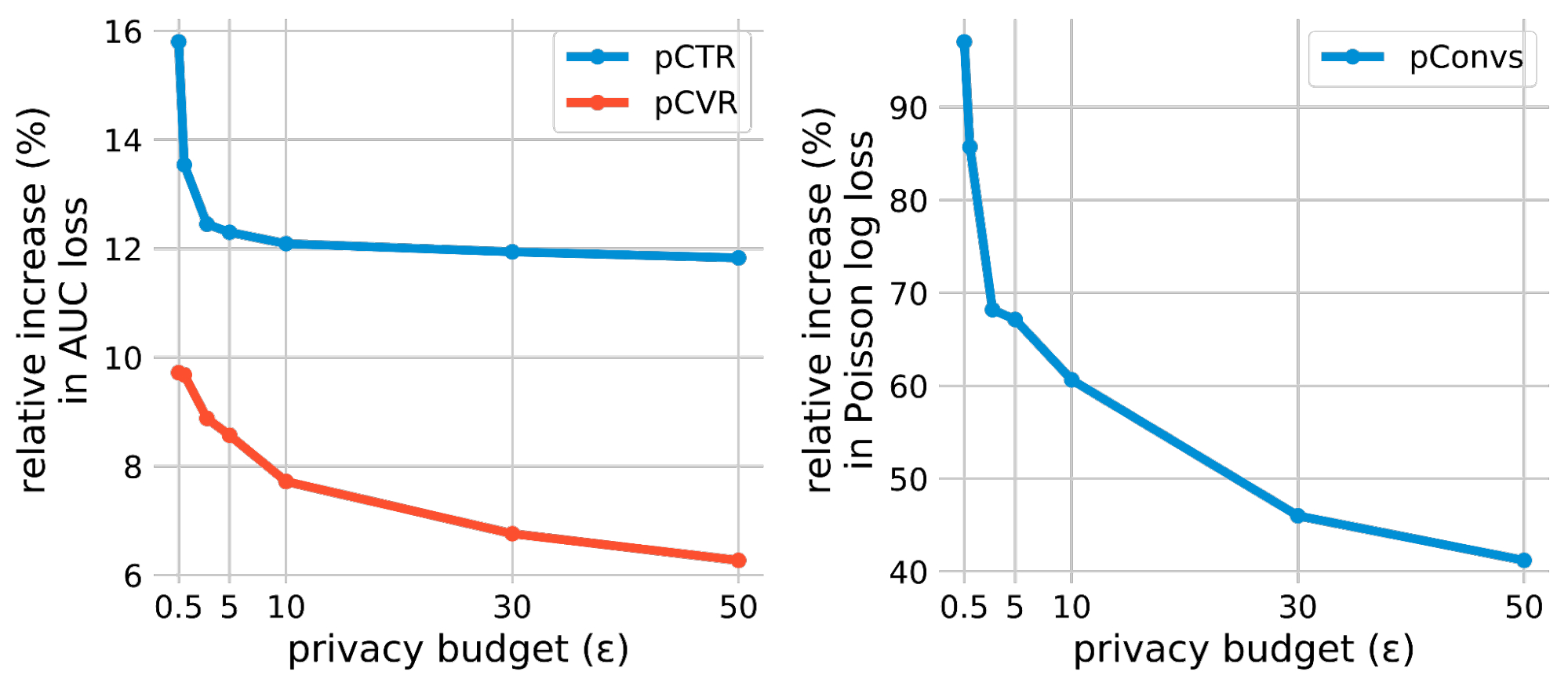DP-SGD results on three ads prediction tasks. The relative increase in loss is computed against the non-private baseline (i.e., ε = ∞) model of each task.

## Improved Privacy Accounting

Privacy accounting estimates the privacy budget (ε) for a DP-SGD trained model, given the Gaussian noise multiplier and other training hyperparameters. Rényi Differential Privacy (RDP) accounting has been the most widely used approach in DP-SGD since the original paper. We explore the latest advances in accounting methods to provide tighter estimates. Specifically, we use connect-the-dots for accounting based on the privacy loss distribution (PLD). The following figure compares this improved accounting with the classical RDP accounting and demonstrates that PLD accounting improves the AUC on the pCTR dataset for all privacy budgets (ε).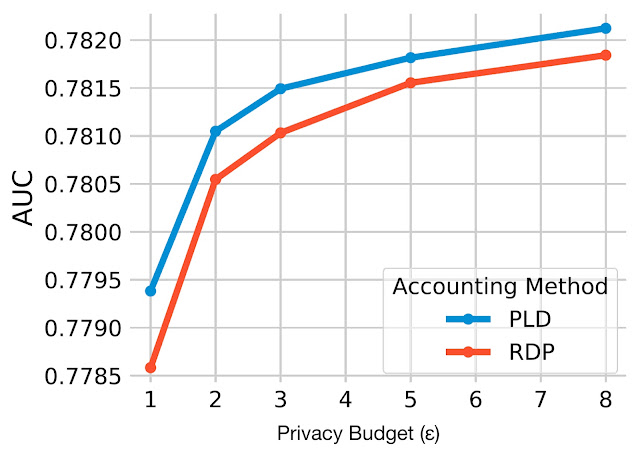## Large Batch Training

Batch size is a hyperparameter that affects different aspects of DP-SGD training. For instance, increasing the batch size could reduce the amount of noise added during training under the same privacy guarantee, which reduces the training variance. The batch size also affects the privacy guarantee via other parameters, such as the subsampling probability and training steps. There is no simple formula to quantify the impact of batch sizes. However, the relationship between batch size and the noise scale is quantified using privacy accounting, which calculates the required noise scale (measured in terms of the standard deviation) under a given privacy budget (ε) when using a particular batch size. The figure below plots such relations in two different scenarios. The first scenario uses fixed epochs, where we fix the number of passes over the training dataset. In this case, the number of training steps is reduced as the batch size increases, which could result in undertraining the model. The second, more straightforward scenario uses fixed training steps (fixed steps).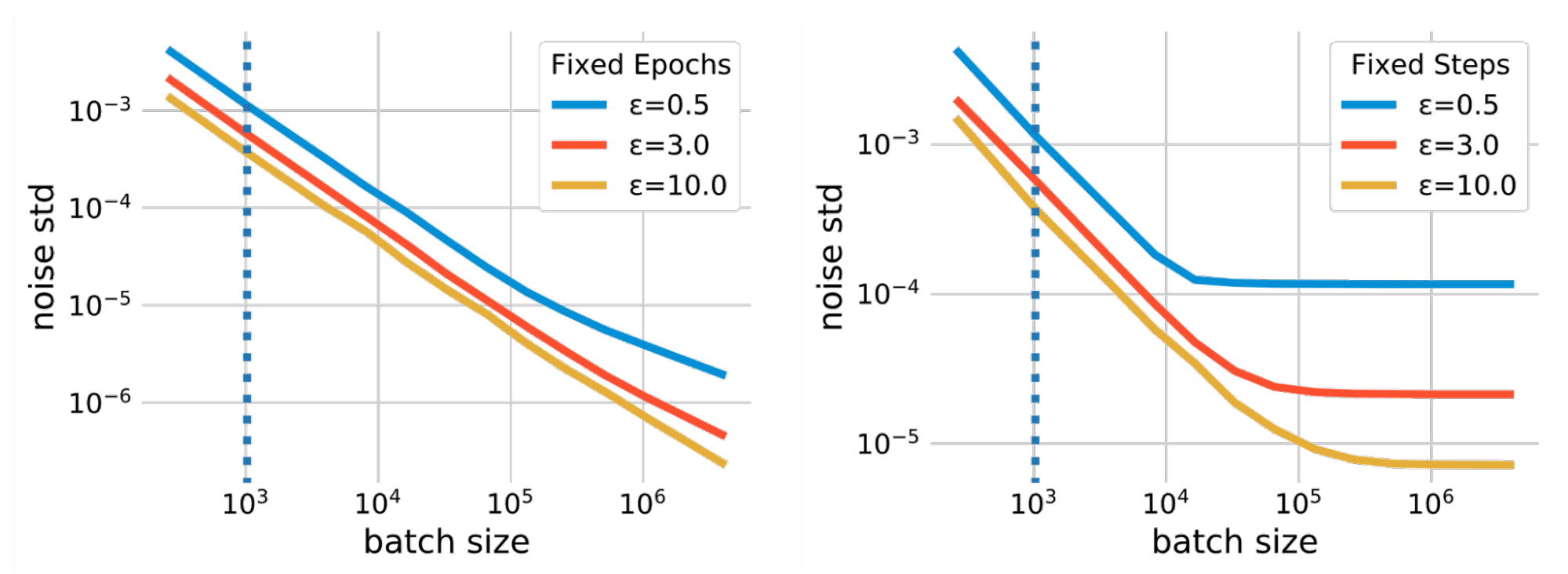The relationship between batch size and noise scales. Privacy accounting requires a noise standard deviation, which decreases as the batch size increases, to meet a given privacy budget. As a result, by using much larger batch sizes than the non-private baseline (indicated by the vertical dotted line), the scale of Gaussian noise added by DP-SGD can be significantly reduced.

In addition to allowing a smaller noise scale, larger batch sizes also allow us to use a larger threshold of norm clipping each per-example gradient as required by DP-SGD. Since the norm clipping step introduces biases in the average gradient estimation, this relaxation mitigates such biases. The table below compares the results on the Criteo dataset for pCTR with a standard batch size (1,024 examples) and a large batch size (16,384 examples), combined with large clipping and increased training epochs. We observe that large batch training significantly improves the model utility. Note that large clipping is only possible with large batch sizes. Large batch training was also found to be essential for DP-SGD training in Language and Computer Vision domains.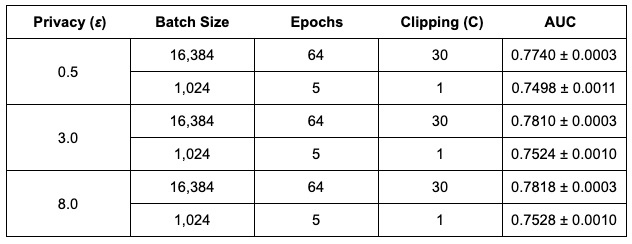The effects of large batch training. For three different privacy budgets (ε), we observe that when training the pCTR models with large batch size (16,384), the AUC is significantly higher than with regular batch size (1,024).

## Fast per-example Gradient Norm Computation

The per-example gradient norm calculation used for DP-SGD often causes computational and memory overhead. This calculation removes the efficiency of standard backpropagation on accelerators (like GPUs) that compute the average gradient for a batch without materializing each per-example gradient. However, for certain neural network layer types, an efficient gradient norm computation algorithm allows the per-example gradient norm to be computed without the need to materialize the per-example gradient vector. We also note that this algorithm can efficiently handle neural network models that rely on embedding layers and fully connected layers for solving ads prediction problems. Combining the two observations, we use this algorithm to implement a fast version of the DP-SGD algorithm. We show that Fast-DP-SGD on pCTR can handle a similar number of training examples and the same maximum batch size on a single GPU core as a non-private baseline.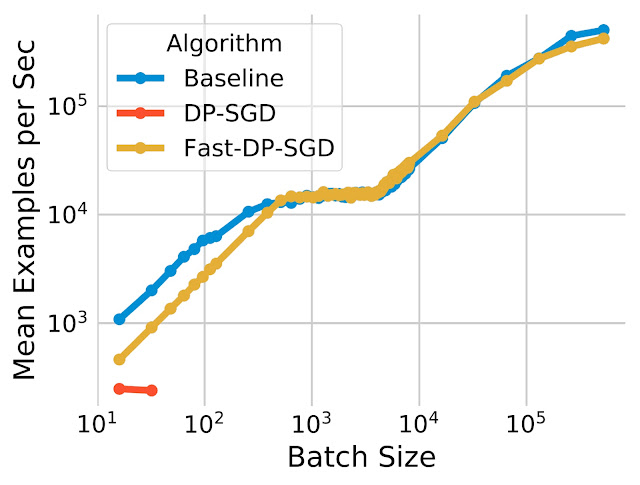The computation efficiency of our fast implementation (Fast-DP-SGD) on pCTR.

Compared to the non-private baseline, the training throughput is similar, except with very small batch sizes. We also compare it with an implementation utilizing the JAX Just-in-Time (JIT) compilation, which is already much faster than vanilla DP-SGD implementations. Our implementation is not only faster, but it is also more memory efficient. The JIT-based implementation cannot handle batch sizes larger than 64, while our implementation can handle batch sizes up to 500,000. Memory efficiency is important for enabling large-batch training, which was shown above to be important for improving utility.

## Conclusion

We have shown that it is possible to train private ads prediction models using DP-SGD that have a small utility gap compared to non-private baselines, with minimum overhead for both computation and memory consumption. We believe there is room for even further reduction of the utility gap through techniques such as pre-training. Please see the paper for full details of the experiments.

## Acknowledgements

This work was carried out in collaboration with Carson Denison, Badih Ghazi, Pritish Kamath, Ravi Kumar, Pasin Manurangsi, Amer Sinha, and Avinash Varadarajan. We thank Silvano Bonacina and Samuel Ieong for many useful discussions.